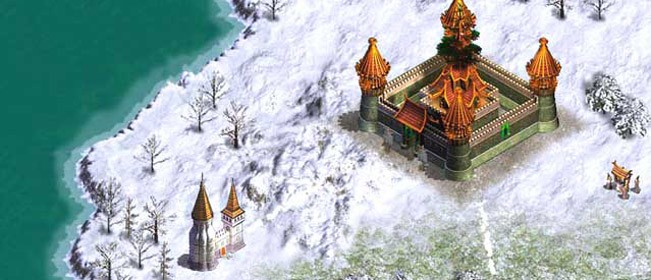﻿

# Warlords IV: Heroes of Etheria### Cheats, Tips & Walkthroughs

#### Other

Warlords 4 hex cheat
Note: You need a hexidecimal editor to use these.

MAKE A BACKUP BEFORE ATTEMPTING.

50=battles fought.
54&58=level (hint: set this to FE (FE is 1) to make you level up fast because your exp is already high, so it will let you get lots of points to spend on skills and buildings).
64=exp -buggy (warlord wont work if you change it, but it does change exp. you cna only view it if you change it)
6c=gate skill
74=merchant skill level
78=defender skill level
7c=antimagic skill level
88=weaponmaster skill level
8c=morale skill level
94=archmage skill level
98=divine magic skill level
9c=rune magic skill level
a4=summoning skill level
a8=necromancy skill level

The values are reversed, so if you are used to hex values, its reversed. its like 256 - hex value is what it will turn out to be, thats why FF=0 and FE=1.

key:
FF=0
FE=1
FD=2
FC=3
FB=4
FA=5
F9=6
F8=7
F7=8
F6=9
F5=10
F4=11
F3=12
F2=13
F1=14
F0=15
EF=16
and so on...

﻿ ﻿